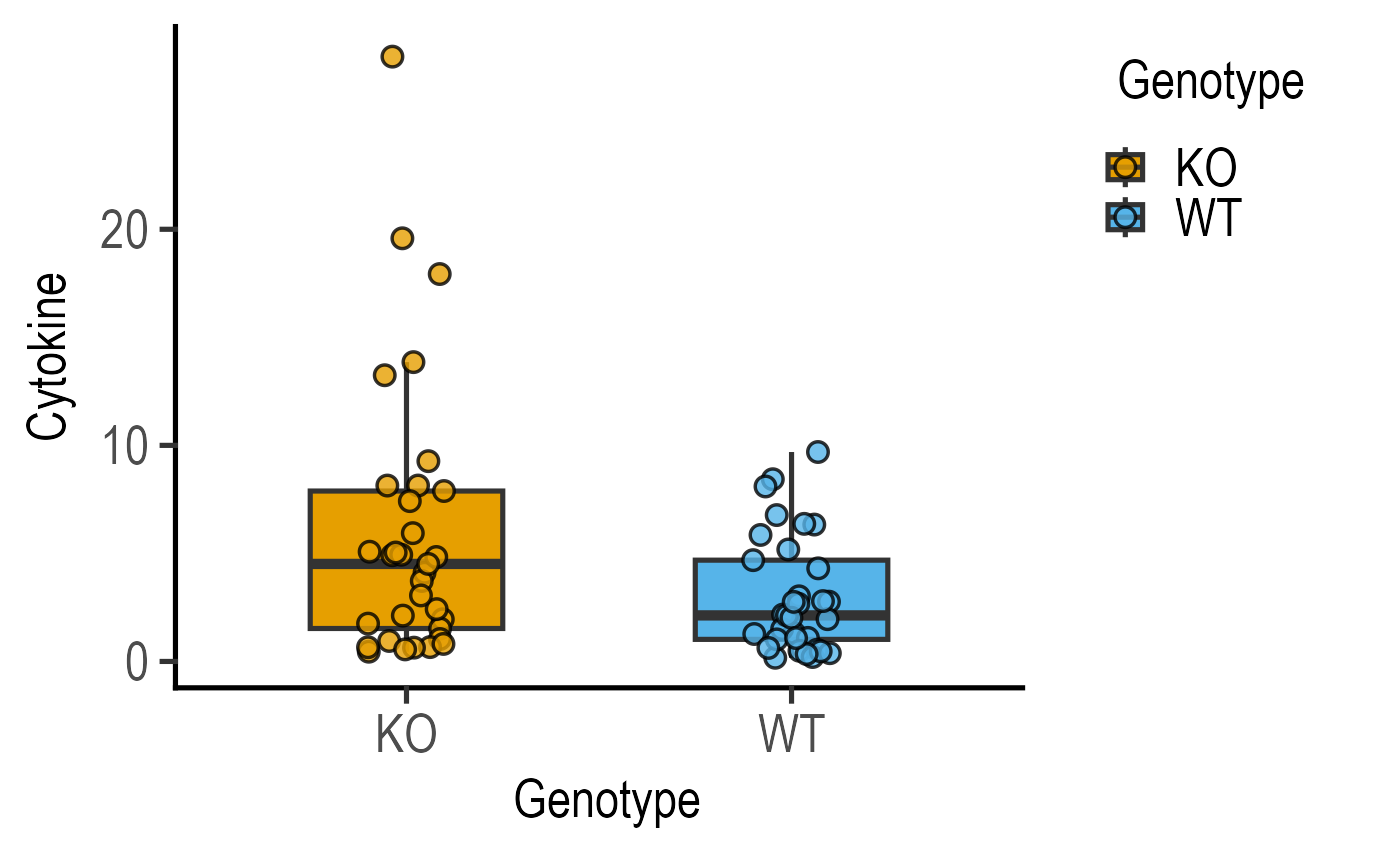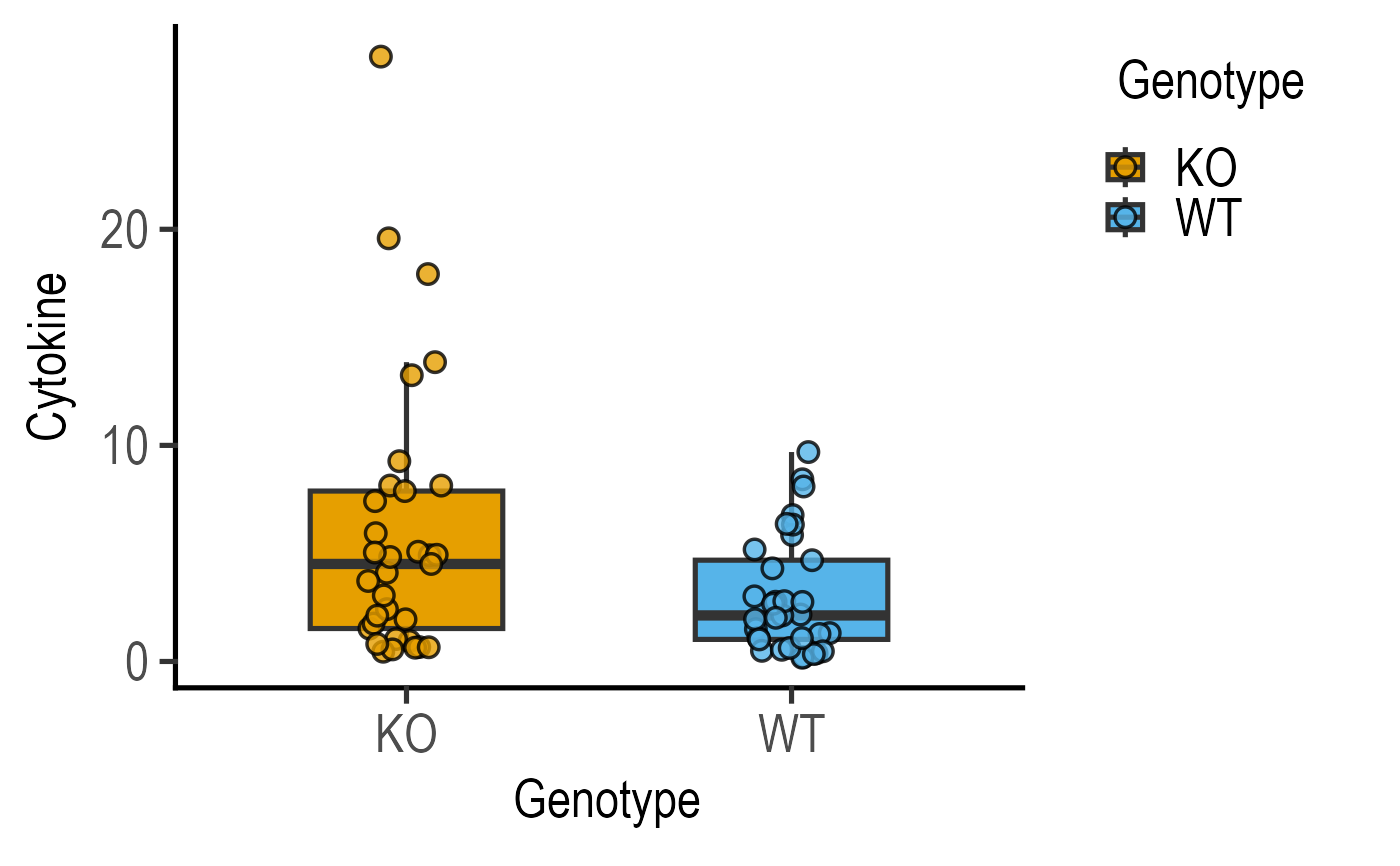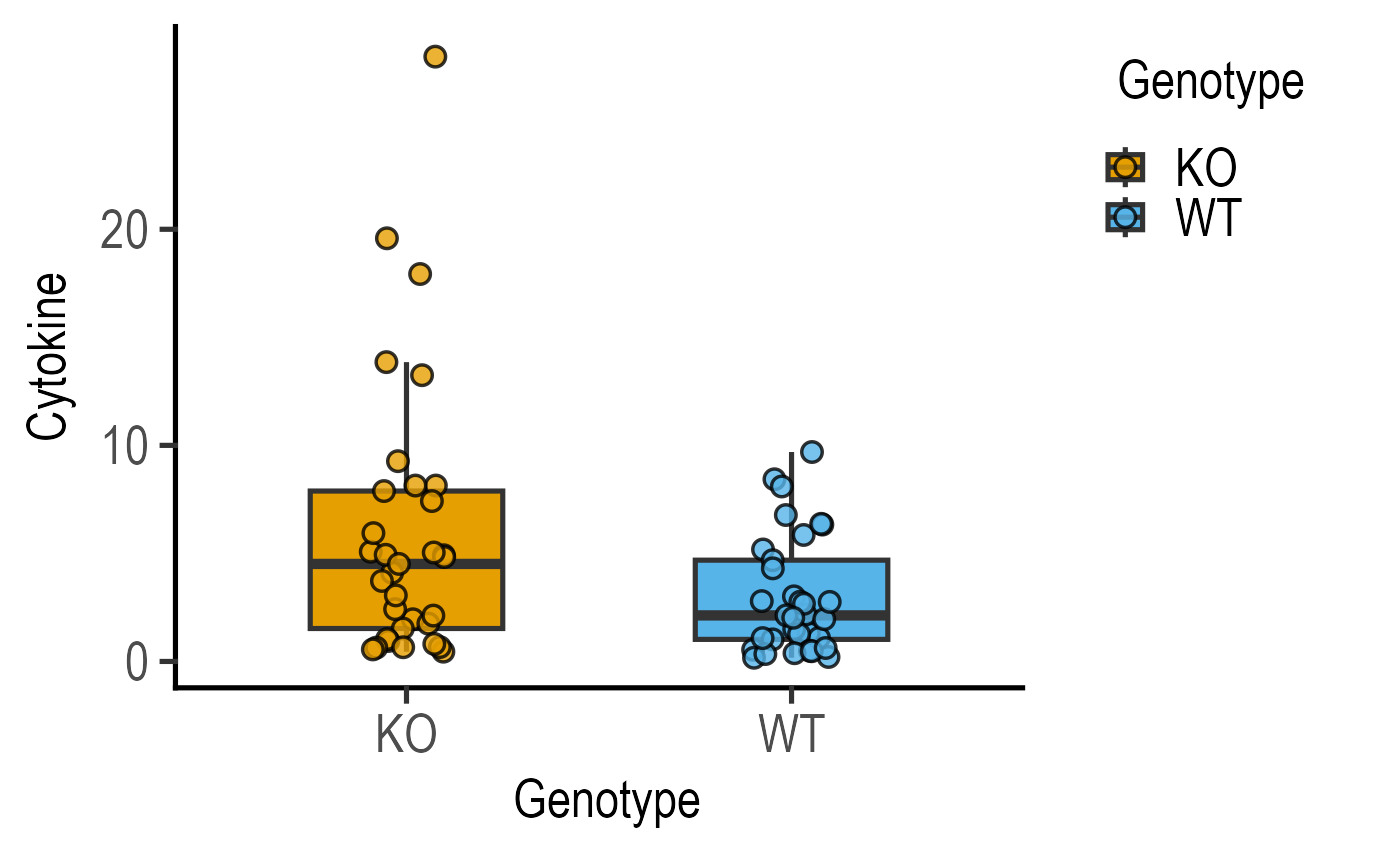This simple function takes in a data table and reorders groups (categorical variables or factors) to be plotted along the X-axis in a user-defined order.

table_x_reorder(data, xcol, OrderX, ...)

## Arguments

data

a data table

xcol

name of column in above data table (provided within quotes) whose levels are to be reordered

OrderX

a vector of group names in the desired order

...

any additional arguments for factor call.

## Value

This function returns a data frame with a selected column converted into factor with reordered levels.

## Details

It uses two base R functions: as.factor to first force the user-selected column into a factor, and factor that reorders levels based on a user-provided vector.

## Examples

#reorder levels within Genotype
new_data <- table_x_reorder(data_t_pratio,
xcol = "Genotype",
OrderX = c("KO", "WT"))
#compare
plot_scatterbox(data_t_pratio,
Genotype,
Cytokine)#with
plot_scatterbox(new_data,
Genotype,
Cytokine)#also works within the plot call
plot_scatterbox(data = table_x_reorder(data_t_pratio,
xcol = "Genotype",
OrderX = c("KO", "WT")),
xcol = Genotype,
ycol = Cytokine)